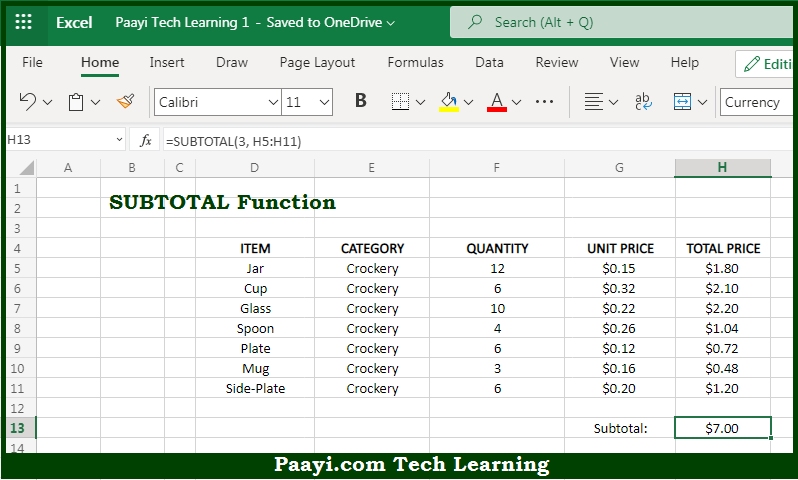# Learn How to Use Microsoft Excel SUBTOTAL Function

Written by | 0 Comments | 646 Views

In this article, you will learn how to use the Microsoft Excel SUBTOTAL function and its prime function in Microsoft Excel. You will also get to know the Microsoft Excel SUBTOTAL function return value and syntax with the help of some examples.

Microsoft Excel SUBTOTAL Function

The main function of Microsoft Excel SUBTOTAL function is to get the subtotal in a list or database. That implies, with the help of the SUBTOTAL function you can able to return an aggregate result of the supplied values. It should be noted that the SUBTOTAL function can return a SUM, AVERAGE, COUNT, MAX, and others you can either include or exclude values in hidden rows using the SUBTOTAL function. So, with the help of the SUBTOTAL function, you can able to get the subtotal of a given list or database.

Return Value of SUBTOTAL Function

The return value will be the specific subtotal.

Syntax of SUBTOTAL Function

=SUBTOTAL(function-num, ref1, [ref2], ...)

Where the arguments:

• number: This is the number that specifies which function to use in calculating subtotals within a list.
• ref1: This is the named range or reference provided to the SUBTOTAL function.
• ref2: This is another named range or reference provided to the SUBTOTAL function (optional).

How to Use Microsoft Excel SUBTOTAL Function?So we know that Microsoft Excel SUBTOTAL function you can able to get the subtotal in a list or database. That implies, with the help of the SUBTOTAL function you can able to return an aggregate result of the supplied values. It should be noted that the SUBTOTAL function can return a SUM, AVERAGE, COUNT, MAX, and others you can either include or exclude values in hidden rows using the SUBTOTAL function. So, with the help of the SUBTOTAL function, you can able to get the subtotal of a given list or database.